"Similar" Division
by Mineyuki Uyematsu and Michael Reid
"Divide a square into two congruent pieces" has an infinite number of solution.  "Congruent" can be regarded as one special case of "similar."  Other shapes may have unique dissections, if certain rules are followed.  Here are the rules:

The Rules
1) Divide a shape into n similar pieces
2) Among n pieces, some or all pieces may be congruent.
3) Turned-over shapes are permitted.
4) Fractal cuts are NOT allowed.  Also, each shape must be solidly connected.

Here is a sample problem -- a triangle with a hole.  Divide the first triangle into two similar shapes.  Divide the second triangle into three similar shapes.  If you are stumped, the answer is here.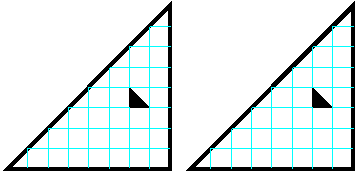Divide into two or three similar shapes. Answer.
In constructing problems, I insisted that upon "unique" solutions.  Unfortunately, several had multiple solutions.Michael Reid made a fantastic series of similar dissection puzzles
mike1, mike2, mike3, mike4, and mike5.

All the problems below have unique solutions, I believe.  Try them!Divide the above figures into TWO similar shapes.Divide the above figures into THREE similar shapes.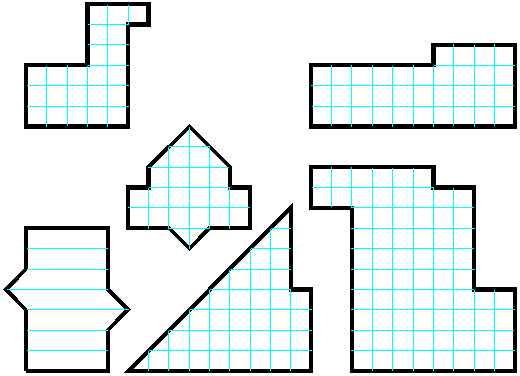Divide the above figures into FOUR similar shapes.

[Many thanks, Mine.  The below figures are similar puzzles I've presented.]  [Here are solutions to all puzzles, by michael reid]
Here's a figure discovered by Gwen Roberts.  Divide it into 5 similar shapes. It's very hard.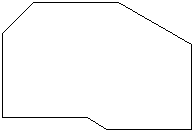Divide into 5 similar shapes.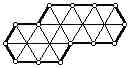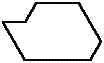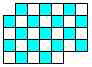Divide each of these figures into two similar shapes.  Answer.

Divide the following figure into five similar shapes.  I drew this with Isisdraw(free).  Solvers were Juha Saukkola, michael reid, and Juraj Lorinc.  I'd be interested in other puzzles of this type, especially when all five shapes are a different size.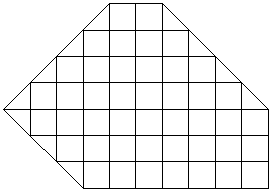Divide into five similar shapes.  Answer.

Here's a puzzle from Rodolfo Kurchan, editor of Puzzle Fun.  The L-tromino is easily divided into four pieces of the same shape.  The classic solution is below.  Can you find a different solution?  Hint: same shape, but different sizes. Answer and solvers.Divide the L Tromino into four similar shapes, in a different way.